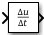# Derivative

Compute approximate continuous derivative of continuous input signal with respect to time

•Libraries:

## Description

The Derivative block approximates the continuous derivative of the continuous input signal u with respect to the simulation time t. Use the Derivative block when you need to compute the derivative for a differentiable signal that has continuous sample time.

Improper use of the Derivative block can lead to inaccuracies in simulation results. When possible, consider these alternatives to the Derivative block:

• Rearrange the system equations to solve for an integral instead of a derivative. Then, structure the model based on the new equations so that the software integrates using blocks such as the Integrator block instead of differentiating.

For an example, see Best-Form Mathematical Models.

• When a Transfer Fcn block also acts on the input or output signal of the Derivative block, implement the derivative for the signal by adding a zero in the transfer function instead.

• To compute the finite difference, or difference quotient, for a discrete signal in a discrete system, use the Discrete Derivative block.

When you must use the Derivative block, use the block with only differentiable input signals that have continuous sample time.

• The software issues a warning when the signal connected to this port has discrete or fixed-in-minor sample time. (since R2023b)

• While the software does not issue a warning when the input signal has constant sample time, tuning the signal value introduces a discontinuity in the input signal. Differentiating across these discontinuities creates spikes in the output signal.

• To compute the finite difference, or difference quotient, for a discrete signal in a discrete system, use the Discrete Derivative block.

### Ensure Accuracy of Continuous Derivative Approximation

Even when used in an appropriate context, the accuracy of the Derivative block output signal depends on the step size and might depend on the dynamics of the rest of the system. When you use the Derivative block, you must ensure that accuracy of the block output signal satisfies your requirements.

Use the Max step size parameter value to prevent a variable-step solver from taking steps that are too large to satisfy your accuracy requirements. To choose an appropriate value for the maximum step size, you might need to run several simulations.

In variable-step simulations, the solver determines the size of the each major time step by monitoring the error in calculations of continuous states throughout the model. When the error in the calculated value for a continuous state exceeds the specified tolerance levels, the solver reduces the step size until the error falls within tolerance.

The Derivative block does not have any continuous states, so the solver cannot adjust the step size to ensure that the Derivative block calculations satisfy tolerance requirements. If the solver takes a large time step when the input signal value changes rapidly, the block might produce an unexpected output value.

## Ports

### Input

expand all

The input signal must be real and can have scalar or vector sample values. Use the Derivative block to approximate the continuous derivative for only signals that have continuous sample time.

Data Types: `double`

### Output

expand all

The Derivative block approximates the continuous derivative of the input signal with respect to time and provides the result as a continuous signal. The output signal dimensions and complexity match the dimensions and complexity of the input signal, which must be real.

Data Types: `double`

## Parameters

expand all

To edit block parameters interactively, use the Property Inspector. From the Simulink® Toolstrip, on the Simulation tab, in the Prepare gallery, select .

Exact linearization for a continuous derivative is difficult because no state-space representation exists for the dynamic equation $y=\stackrel{˙}{u}$. The software approximates the linearization of the Derivative block by adding a pole to the system to create a transfer function that has the form $s/\left(c\ast s+1\right).$. The pole also acts as a low pass filter with a cutoff frequency determined by this parameter value, which reduces the effect of noise.

As a best practice, specify this parameter value as $\frac{1}{{f}_{b}}$, where ${f}_{b}$ is the cutoff frequency in radians per second for the low pass filter that results from the addition of the pole. Choose a cutoff frequency greater than or equal to the Nyquist rate or frequency for the system. The default parameter value `inf` corresponds to a cutoff frequency of `0`.

#### Programmatic Use

To set the block parameter value programmatically, use the `set_param` function.

To get the block parameter value programmatically, use the `get_param` function.

 Parameter: `CoefficientInTFapproximation` Values: `inf` (default) | finite positive scalar number Data Types: `char` | `string`

Example: `set_param("MyModel/Derivative","CoefficientInTFapproximation","0.001")` configures the Derivative block named `Derivative` in the model `MyModel` to use the coefficient `0.001` in the transfer function used for approximate linearization of the block. This coefficient value results in a cutoff frequency of `1000` radians per second.

## Block Characteristics

 Data Types `double` Direct Feedthrough `yes` Multidimensional Signals `no` Variable-Size Signals `no` Zero-Crossing Detection `no`

## Algorithms

The Derivative block computes the numerical difference $\Delta u/\Delta t,$ to approximate the continuous derivative $\frac{du}{dt},$, where $\Delta u$ is the change in the input signal value and $\Delta t$ is the size of the major time step the solver took to reach the current simulation time. The accuracy of the continuous derivative approximation depends on the rate of change for the input signal and the step size.

The initial output value for the Derivative block is always `0`. Taking the initial output value into consideration, this equation represents the precise relationship between the block input and output signals.

`$y\left(t\right)=\frac{\Delta u}{\Delta t}=\frac{u\left(t\right)-u\left({T}_{previous}\right)}{t-{T}_{previous}}|t>{T}_{previous},$`
• t is the current simulation time.

• ${T}_{previous}$ is the time of the last major time hit in the simulation.

## Version History

Introduced before R2006a

expand all